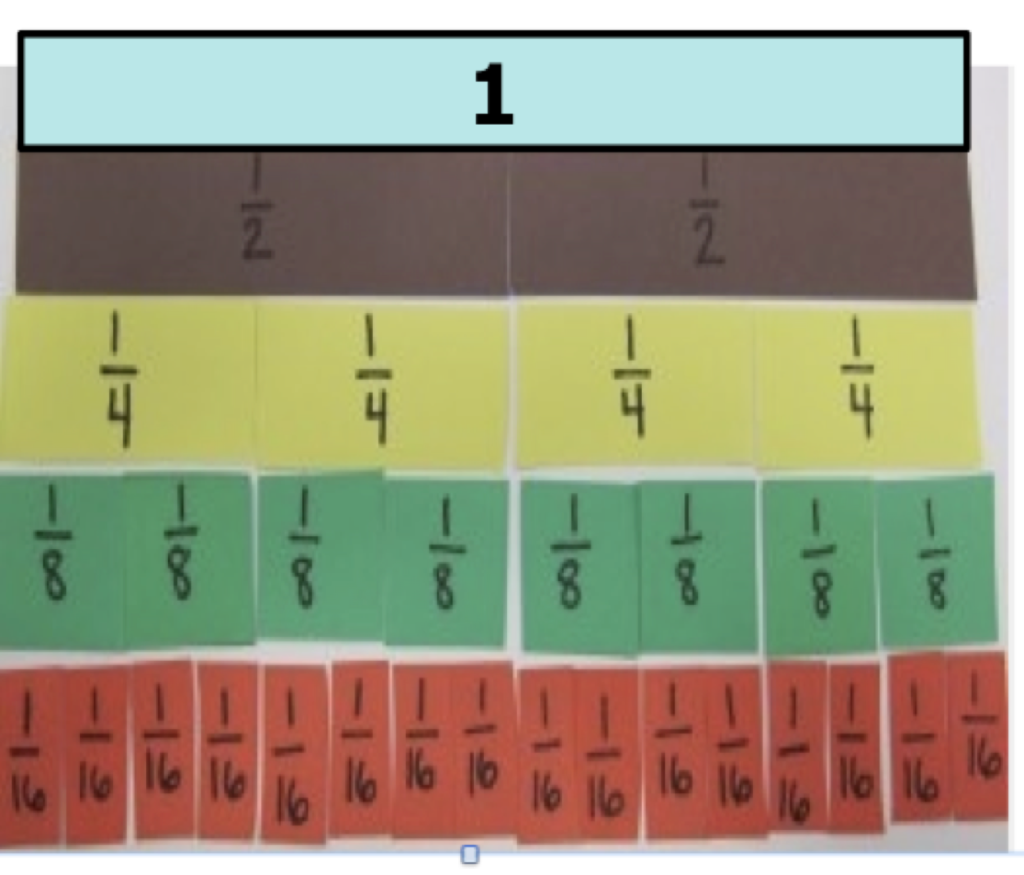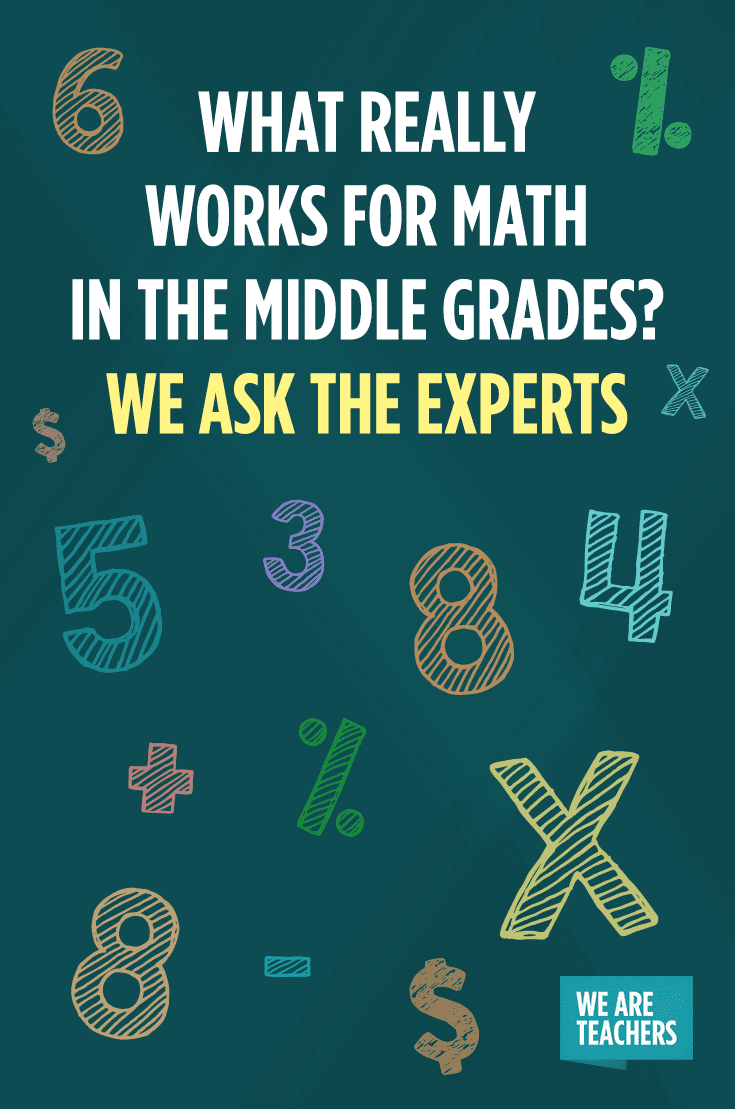# What Really Works for Math in the Middle Grades? We Ask the ExpertsOne topic that comes up again and again on our WeAreTeachers Helpline is teaching tough math concepts. As students move into upper elementary and middle school, teachers aren’t always sure how to teach some of the more complex mathematical concepts. That’s why we jumped at the chance to connect with Sherry Parrish and Ann Dominick, the experts behind the HMH Math Solutions Number Talks series, to ask some of your toughest Helpline questions.

## 1. “It seems by the fifth grade, students’ understanding of number sense should be firmly established. Why is it important to continue to teach this concept?” —Courtney H.

The best definition we know of number sense is from the book Number Sense by Fennell and Landis.

Number sense is “… an awareness and understanding about what numbers are, their relationships, their magnitude, the relative effect of operating on numbers, including the use of mental mathematics and estimation.” (1994)

As students move from understanding tens to hundreds, to thousands and beyond, they have to build an understanding of this hierarchy of numbers. As they transition into each area of number, they have to again build number sense. The same is true when moving into rational and irrational numbers. Students need to develop a deep understanding of this realm of number. Number sense is continually evolving as students transition into each grade level.

A great way to help build number sense is to have 5- to 15-minute classroom conversations around purposeful problems that students solve mentally. When we ask students to think about a computation problem mentally, it encourages them to think about the numerical relationships.

Consider the problem 3/4 + 5/8. How could you solve this problem mentally without using the standard U.S. algorithm? A couple of ways we’ve seen students approach this problem are:

1) Decomposing to Make a Whole

3/4 + 5/8

= 3/4 + (2/8 + 3/8)

= (3/4 + 1/4) + 3/8

= 1 + 3/8

= 1 3/8

2) Compensating to Make a Friendly Number

3/4 + 5/8

(3/4 + 1/4) + 5/8

= 1 + 5/8

= 1  5/8

1  5/8 – 1/4 = 1 3/8

By being thoughtful about the tasks and questions we pose to students, we can help them continue to develop and use number sense.

## 2. “How can I come up with sample math problems that mean something to my students and will also meet the needs of a diverse group of learners?” —Gretchen V.

Posing an isolated problem and asking students how they might solve it provides access from various perspectives. For example, if we pose the problem 49 + 49, some students might add by place value and combine the tens and ones, while others might round each up to 50 and then subtract the extra 2 they added when they rounded two 49s to 50. Presenting problems and asking students how they could solve them allows students to focus on the mathematical relationships that can help them find solutions.

Another option is to incorporate a series of problems to help students consider and use relationships between problems. For example, we could present the following string to help students think about how factors can be decomposed into smaller factors when multiplying.

2 x 25

4 x 25

6 X 25

12 x 25

This purposeful progression encourages students to begin with easier problems and then use those problems to solve additional problems. Students might use what they knew from solving 2 x 25 or 4 x 25 or 6 x 25 to solve 12 x 25.

(4 + 4 + 4) x 25

(6 + 6) x 25

(2 + 4 + 6) x 25

(3 x 4) x 25

(2 x 6) x 25

## 3. “I need help making math more interesting for my students. It is a difficult subject for my kids and they struggle. How can I keep my students engaged?” —Mark D.

One way to make math more accessible and engaging is to start your math time with a Number Talk. Teachers and students cannot say enough about how much they enjoy this part of their day! The beauty of a Number Talk—which is a 5- to 15-minute classroom conversation around purposeful problems that students solve mentally—is that students have the chance to develop their own strategies, share their ideas and learn from one another. The problems that students solve build on what they already know, so everyone has access to the mathematics, and because the problems can be solved in a variety of ways, students are challenged to think of as many ways as possible to find a solution. There’s no “down” time! When teachers use a turn-and-talk strategy, students all have a chance to share their thinking, and they verify their answers by using different strategies and coming up with the same answer. Students actually enjoy math when they realize that it makes sense and they are engaged in finding solutions.

## 4. “My kids are having a hard time understanding ‘simplest form’ when it comes to fractions. I couldn’t get the wording right. Any good ideas of manipulatives out there for older kids?” —Arlene N.

Often when we teach students to find the simplest form, we tell them to divide the numerator by a specific number and then divide the denominator by the same number. For instance, to change 6/8 to simplest form we might say “divide the numerator and the denominator by 2.” What we should focus on instead is that we are dividing 6/8 by 2/2, or 1, which is why 6/8 is equal to 3/4. This procedure is actually based on the identity property of division, which states that any number divided by 1 is still that number. This is the big idea that we want students to investigate to build a deeper understanding of fractions and simplest form.

One way we can help students explore these big ideas is to use purposefully selected fractions as a context for a short classroom conversation. Consider the following area model using halves, fourths, eighths and sixteenths.First ask students, “What are some things you notice?” In our interactions with students, we often hear: the smaller the denominator the larger the piece, the larger the denominator the smaller the piece, it takes two 1/4s to make 1/2, etc. As students begin to offer other ways to make a half, list their ways (1/2, 2/4, 4/8, 8/16) and ask, “What do you notice?” By approaching simplest form and equivalence in this way, students are developing fractional reasoning and you can begin conversations about simplest form and equivalence.

## 5. “I’m looking for ideas for student-driven activities that we can do for comparing and ordering decimals. Any ideas?” — Jessica B.

One way to help students understand comparing and ordering decimals is to give them several decimal numbers to consider and then think about how they would order them. Ask students to put their fists on their chests and put their thumbs up when they have an answer to how they would order the decimal number from least to greatest. After students share their answers, they discuss why they put the decimals in the order they chose. Defending and discussing their solutions gives students an opportunity to think and reason about using place value to understand the magnitude of the decimal numbers and to reason about equivalence.

A number line can be a good tool. Using a number line that extends beyond 0-1 can help uncover some student misconceptions and deepen students’ understanding. For instance, if students are asked to put 0.5 on a number line that extends from 0 to 1, many students can be successful. When you use a number line that goes from 0 to 2, however, many students will put 0.5 in the middle of the number line at the number 1 because it is half of the number line. Giving students opportunities to discuss and defend their thinking is a critical part of using number talks and helping students to make sense of mathematics.

Like these answers? Check out our giveaway with all of our favorite resources for teaching math in the middle grades. Enter today.

The Math Solutions Number Talks series can help you have conversations about math that will deepen students’ understanding of tough concepts and boost their confidence in math. For more resources on how to implement Number Talks in your classroom, check out  Number Talks: Whole Number Computation and Number Talks: Fractions, Decimals, and Percentages.